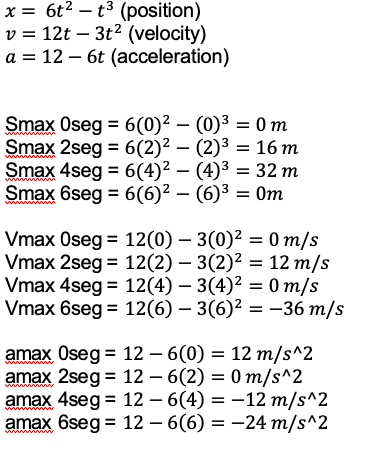# Rectilineal movement of particle

Krokodrile
Homework Statement:
consider particles with a motion given by: x= 6t^2-t^3 and make a excel graphic. Obtain maximum velocity, maximum aceleration and maximun displaiment
Relevant Equations:
d/dv
I tried to resolve this problem with youtube tutorials help, but, i have a many wrong results. The teacher says that the problem requires critics points, so i know that for that points i need a second grade ecuation, but i don't know how interpreter that points. My results:PD: I don't know how make a MRU graphic in excel.

Homework Helper
Gold Member
2022 Award
It is not clear to me whether you are asked to obtain the answers by means of a graph, or whether you are supposed to use algebra and the graph is extra.
For each of the three maxima you are asked for, you have the right answers in your samples. But that is unreliable - you have no easy way to see whether higher values might occur elsewhere.
To get these algebraically you have to do the following:
- find local extrema by taking the derivative to be zero; e.g. for max displacement look for when its derivative (the velocity) is zero. 12t-3t2=0, t=4, x=32.
- check which extrema are maxima, not minima
- check whether a boundary value (in this case only t=0) gives a greater value than the local maxima.

Last edited:
Krokodrile
It is not clear to me whether you are asked to obtain the answers by means of a graph, or whether you are supposed to use algebra and the graph is extra.
For each of the three maxima you are asked for, you have the right answers in your samples. But that is unreliable - you have no easy way to see whether higher values might occur elsewhere.
To get these algebraically you have to do the following:
- find local extrema by taking the derivative to be zero; e.g. for max displacement look for when its derivative (the velocity) is zero. 12t-3t2=0, t=4, x=32.
- check which extrema are maxima, not minima
- check whether a boundary value (in this case only t=0) gives a greater value than the local maxima.
I have to make three extra graphics, i suposed that is time vs velocity, aceleration and position.
yes, i see in a basic hand graphic that local extreme its maxima. I have a question about the x=32, ¿how? i know how obtain t=4 but not that 32.
The teacher mencionated that something curious is happening with the particle trayectory, so i guess that i can see that in the excel graphic, but i don't know how interprete it.